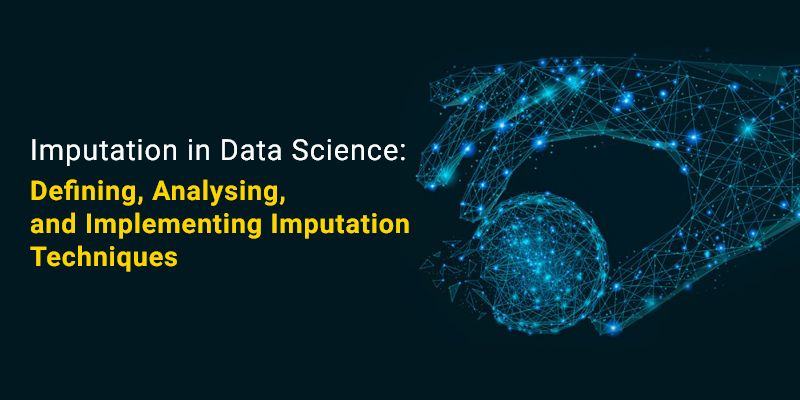Though various evolving technologies exist in the digital world, data science is the most demanding, unique, fast-growing, and sought-after career. Big Data offers quick solutions to problems for businesses, non-profits, and governmental organizations across all industries. An almost limitless data source can be arranged, examined, and used for several purposes.

Could you imagine how a company would analyze purchase history to devise a marketing strategy? How can government agencies utilize behavior patterns to develop entertaining community events? How should a non-profit use its marketing budget to maximize the potential of its operations?

The data scientist will utilize all these processes and implement a data-driven approach. The primary work of the data scientist is to collect, organize and evaluate data to aid the people working in every part of the industry.

If you have strong perseverance in becoming a data scientist, you can join the Data Science Course in Chennai, which will help you understand machine learning, deep learning, artificial Neural Networks and Imputation in data science.

In this blog, we shall discuss what Imputation is, Imputation in data science, imputation techniques, and what is Imputation in machine learning.

## What is Imputation?

Imputation techniques are used in data science to replace missed data with substitution values. The main purpose of this replacement process is to retain the data dataset.

To better understand imputation and variables, you can join the Data Science Online Course and impart knowledge of data science concepts and learn advanced statistical concepts.

The implementation of imputation techniques is to remove the data from the dataset daily. These methods are employed because it would be impractical to remove data from a dataset each time. Additionally, doing so would substantially reduce the dataset’s size, raising questions about bias and impairing analysis.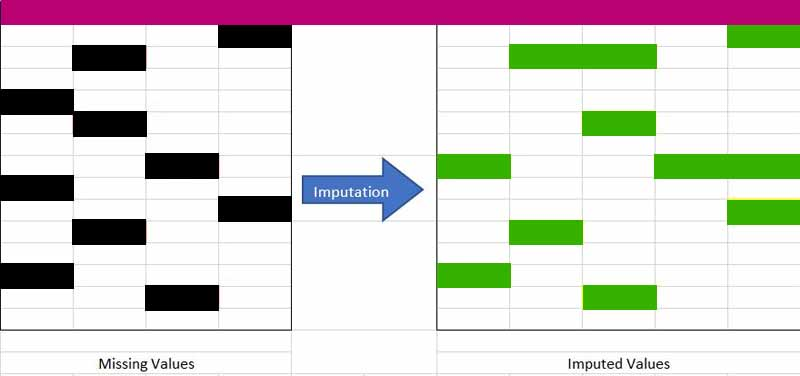Let us understand via image. You can comprehend the missing data on the left table(black) from the above image. We shall fill the missing dataset in the right table(green) without reducing the dataset’s real size. As can be seen, we have increased the column size here using the Imputation strategy (Adding “Missing” category imputation).

## Why is Imputation Important?

Now, you will understand what is Imputation. We will discuss why we should utilize it and the drawback we face if we don’t use it in detail.

We use Imputation because Missing data can cause the below issues:

Imputation in machine learning with the python libraries In the machine learning process, python libraries are widely utilized. The standard python libraries include Scikit-learn, Pandas, TensorFlow, Seaborn, Theano, Keras, etc. Utilizing these libraries led to errors because they did not provide the automatic handling of these missing data.

If you intend to learn python programing language, you can join Python Training in Chennai, which will help you build your career growth because python is a pivotal language used in the development, data science, and software field.

• Distortion in DatasetA significant amount of missing data might modify the variable distribution, changing the value of a specific category in the dataset.
• Affects the Final ModelMissing data can affect the dataset’s statistics and cause the model’s analysis to be invalid.

Another fundamental reason is that “We desire to restore the entire dataset. “Because every piece of information is essential, we typically do this if we don’t want to miss any (or more) data in our dataset.

Secondly, the size of the data set is massive, so if we intend to remove any part, it may significantly impact the final model.

Let’s now analyze and compare the various imputation techniques. But first, it’s important to comprehend the various types of data that make up our dataset.

Now, we shall discuss the four types of data in-depth. The data are:

• Numeric
• Categorical
•  Date-time & Mixed.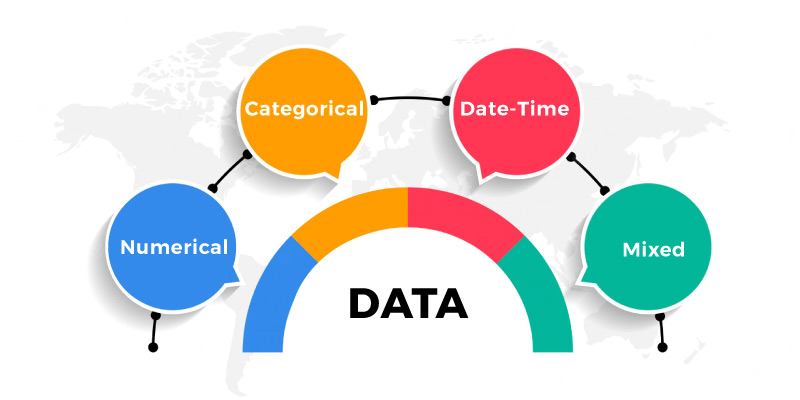## Imputation Techniques

Now we shall move on to learning the main objective of our blog,’ Strategies for Imputation.’ Imputing is a strategy to handle the missing data in the datasets.

Join the Python Online Course, which meticulously designed the course syllabus for learners who intend to learn from the comfort of their homes. So, learners who take this course will get wider career opportunities for working in various fields.

### Numerical variables

• Arbitrary Value Imputation
• Start/End of Distribution Imputation
• Mean/Median/Mode Imputation.
• KNN Imputation.

### Categorical Variables

• Frequent Category imputation

### Both Variables

• Analyze the case completely
• Random Imputation.

### Complete Case Analysis(CCA)

CCA method is utilized explicitly for handling the missing data.

The approach for handling missing data is relatively simple because it eliminates the rows with missing data so that we only consider the rows with complete data or data that are not missing.

In common usage, this technique is sometimes referred to as listwise deletion.

• Assumptions
• Data is MAR(Missing At Random)
• Missing data is entirely drawn from the table.
• Effortless for implementation.
• No Data manipulation is mandated.
• Limitations
• Deleted data can be instructive.
• It may result in a significant amount of data being deleted.
• If a substantial portion of a certain kind of variable is removed from the dataset, it may result in bias.
• The production model won’t know what to do when there are missing data.
• When to Use
• Data is Missing At Random
• Suitable for Numerical, Categorical, and Mixed data.
• Missing data is less than 5% – 6% of the dataset.
• Data doesn’t contain much information and will not bias the dataset.

### Code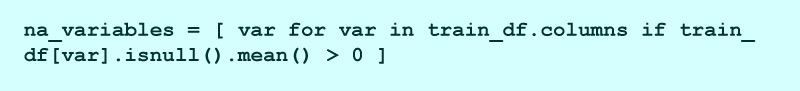## We can see the mean Null values present in these columns data_na = trainf_df[na_variables].isnull().mean()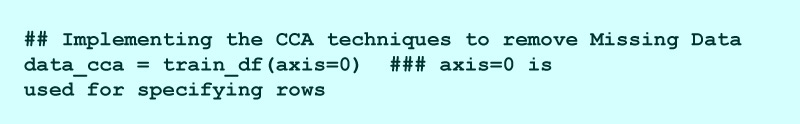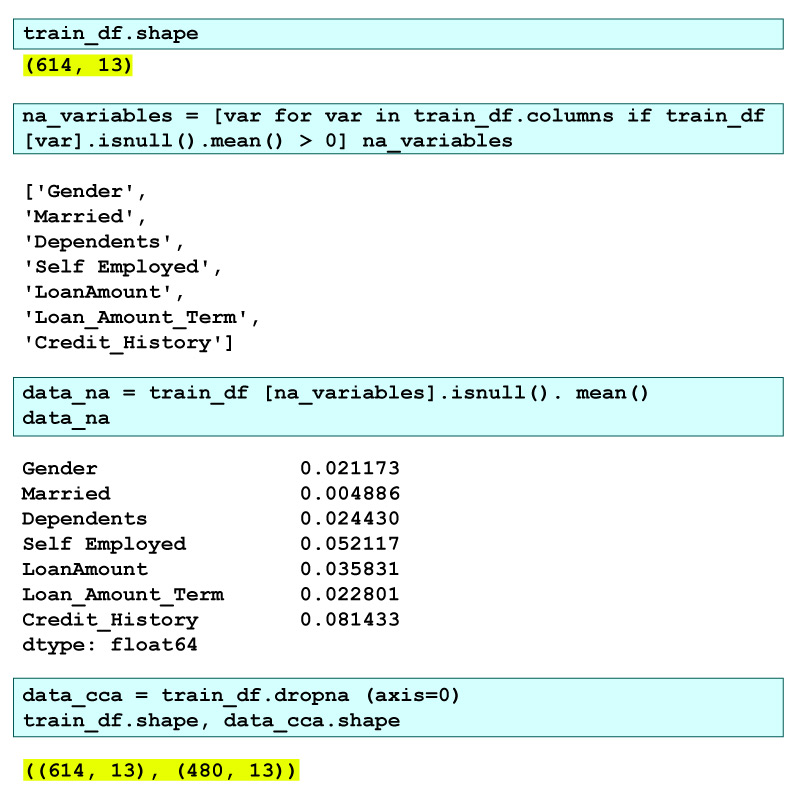Here we can notice the dataset initially had 614 rows and 13 columns, out of which seven had missing data. It means the missing rows are shown by data_na. We see that apart from <Credit_History> & <Self_Employed> all have mean less than 5%.

Therefore, by the Complete Case Analysis, we eliminated the rows with missing data leaving a dataset with only 480 rows. This data reduction, which is about 20%, can lead to many problems in the future.

### Arbitrary Value Imputation

It is another essential technique used in Imputation. With the Arbitrary Value Imputation, we can control both the Categorical and Numerical variables.

According to this method, we gather missing values in a column and allocate them to a new value outside the limits of that column. For numerical & categorical variables, we typically utilize values like:

• 99999999 or -9999999 or
• “Missing” or
• “Not defined.”
• Assumptions
• Data is not Missing At Random.
• The missing data are imputed using an arbitrary value that is not a part of the dataset, the mean, median, or Mode of the data.
• Effortless to implement.
• We can utilize it in production.
• It upholds the importance of “missing values” if it exists.
• The original variable distribution is distorted.
• Arbitrary values can produce outliers.
• Extra effort is needed in choosing the Arbitrary value.
• When to Use
• When data is not Missing At Random (MAR), we can use it.
• Appropriate for All.

### Code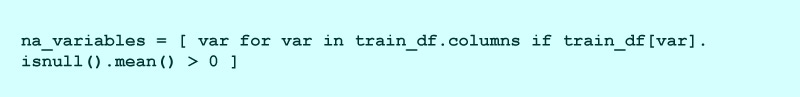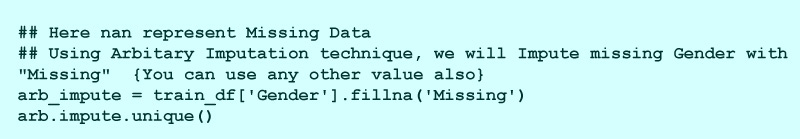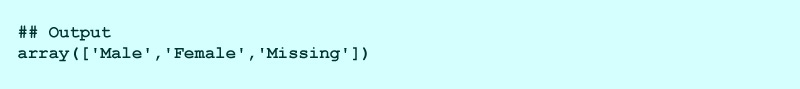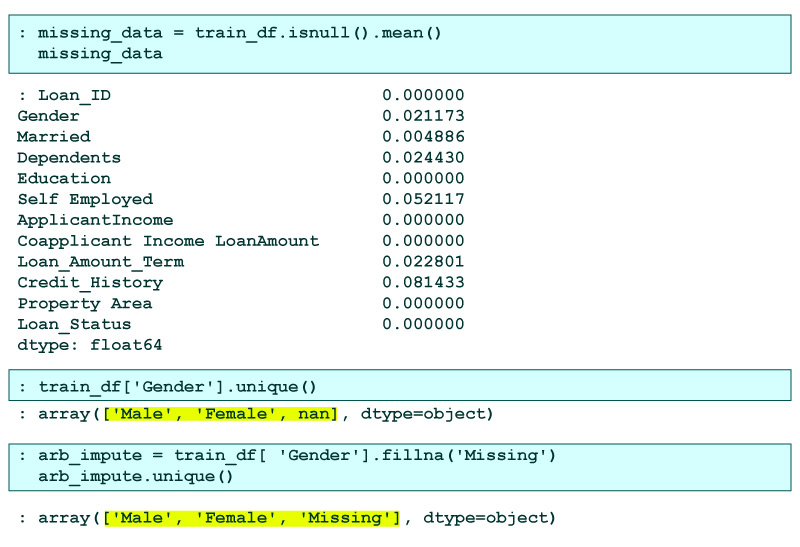### Frequent Category Imputation

Imputing is a strategy to handle missing values in the Frequent Category Imputation. This technique replaces the missing values with the Mode of that column or with the highest frequency. This technique is also named Mode Imputation.

• Assumptions
• Data is missing at random.
• There is a chance that the missing data seems like most of the data.
• Execution is effortless.
• We can receive a complete dataset within a little amount of time.
• We can employ this technique in the production model.
• The distortion will increase as the percentage of missing values increases.
• It could result in a category being overrepresented.
• The original variable distribution is distorted.
• When to Use
• Data is Missing at Random(MAR)
• Missing data is less than 5% – 6% of the dataset.

### Code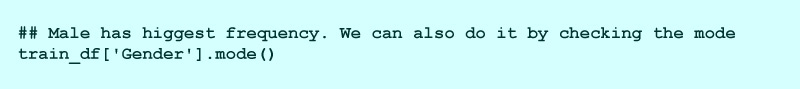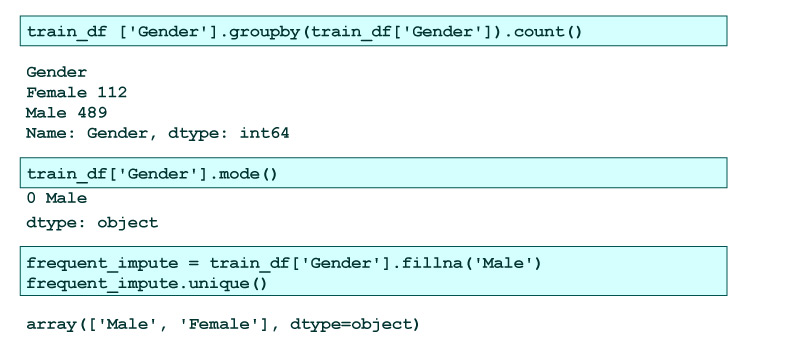Now that you would have understood Imputation in data science and Imputation in machine learning and imputation techniques. So, to learn more about Imputation, you can join Data Science Courses in Bangalore, which will help you have a profound understanding of core concepts in data science, Data Manipulation using Python, Machine Learning Models, and Data Visualization.